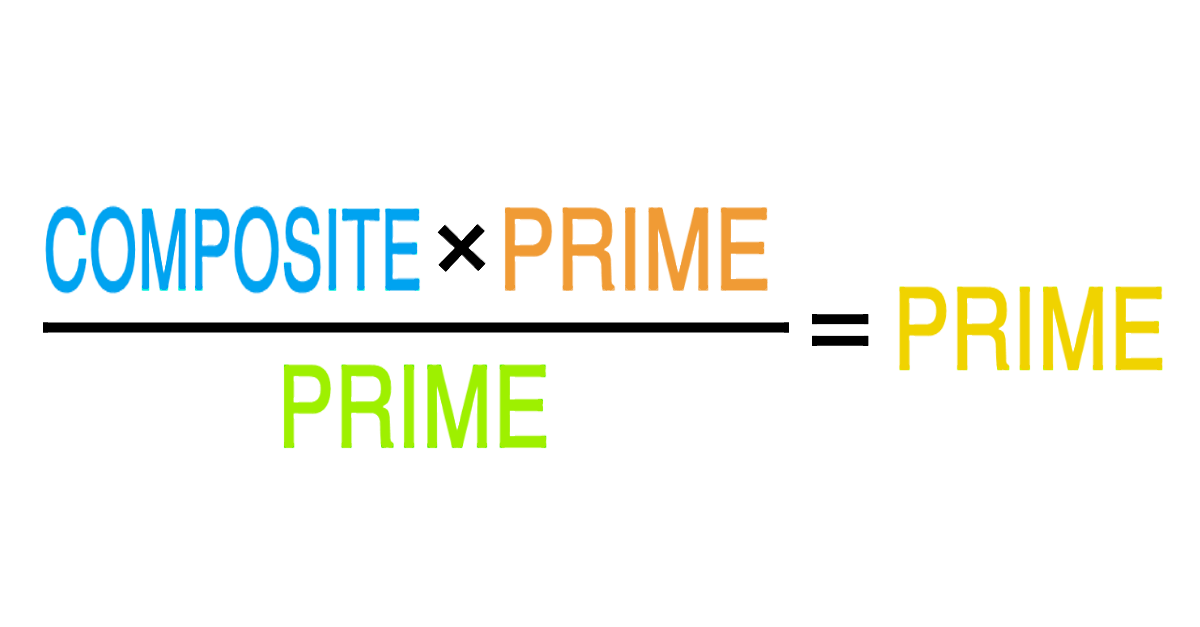# Prime PossibilitiesCan a composite number, times a prime number, divided by another prime number ever equal a prime number?

If "yes," then find three numbers that work.
If "no," then why not?

Clarification: The three prime numbers can be different numbers.

×